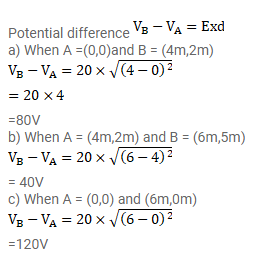# An electric field of 20 N/C exists along the X-axis in space.

Question:

An electric field of $20 \mathrm{~N} / \mathrm{C}$ exists along the $\mathrm{X}$-axis in space. Calculate the potential difference $\mathrm{V}_{\mathrm{B}}-\mathrm{v}_{\mathrm{B}}$ where the points $\mathrm{A}$ and $\mathrm{B}$ are given by,

(a) $A=(0,0) ; B=(4 m, 2 m)$

(b) $A=(4 m, 2 m) ; B=(6 m, 5 m)$

(c) $A=(0,0) ; B=(6 m, 5 m)$.

Do you find any relation between the answers of parts

(a), (b)and (c)?

Solution: